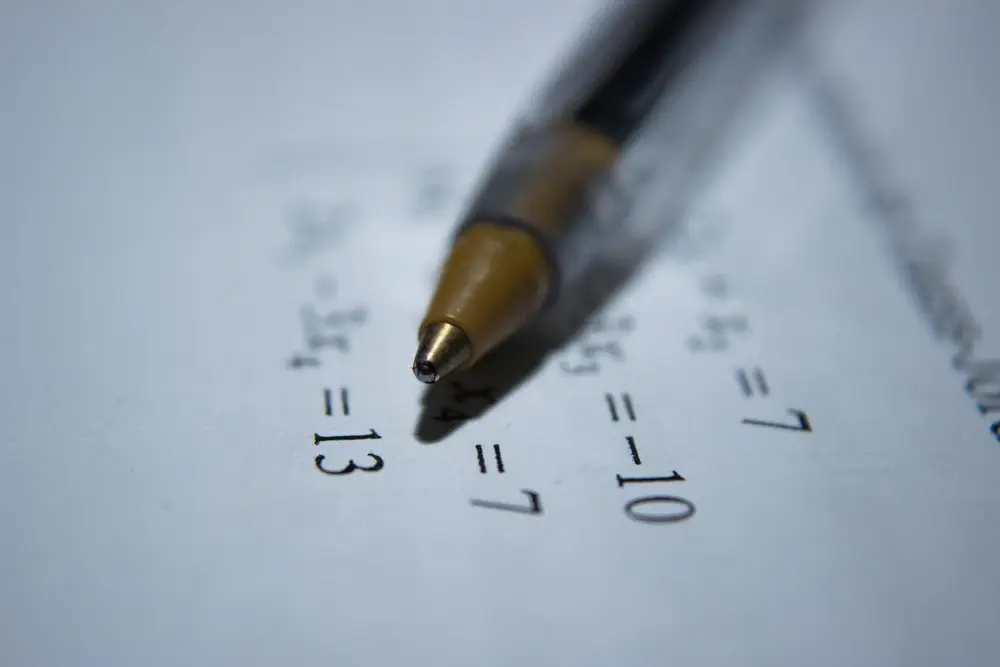# Flow charts

Flow charts show a set of instructions which are to be followed. When you put a value into the start of the flow chart and follow the results you should get a result.

Flow charts are made up of boxes, each with their own function. The shape of the box shows what it is doing.

These boxes are joined together by arrows which shows the direction you have to follow the instructions.

An example of a simple flow chart follows:

If you think of a number and follow the flow chart you would get a new result.

If you think of the number 2, you would:

• add 5 to 2 to get 7
• multiply 7 by 3 to get 21

This means when you put 2 in, you get 21 out.

You can get the same result by using the equation y = 3 ( x + 5 ). Where x is the number you put in and y is the result. As a function, it would be seen as: f(x) = 3 ( x + 5 )

When looking at the equation you can easily change the subject:

• y = 3 ( x + 5 )
• y/3 = x + 5
• y/3 - 5 = x

This means the inverse function is: f-1(x) = x/3 - 5

This can be made into an inverse of a flow chart:

If you think of a number and follow the flow chart you would get a new result.

If you think of the number 21 from the first result, you would:

• divide 21 by 3 to get 7
• subtract 5 from 7 to get 2

The inverse has returned the number back to what the subject used to be.

Algebra

Algebra

Algebra

Algebra

Algebra

Algebra